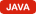# Namespace: Vectors

## Vectors

A factory object containing an helper method to generateVec3D instances.

### Methods

#### <static> newVec3D(x, y, z, isFloat)

Creates an instance ofVec3D, which defines a set of coordinates in a virtual world represented by a MMORoom.
##### Parameters:
Name Type Description
`x` number The position along the X axis.
`y` number The position along the Y axis.
`z` number The position along the Z axis.
`isFloat` boolean Force the coordinates to be treated as floating point numbers (even if integers are passed).
##### Returns:
AVec3D instance.
Type
Vec3D